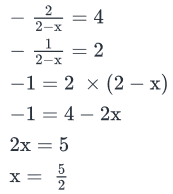Courses

# Test: Linear Equations- 1

## 10 Questions MCQ Test Quantitative Aptitude for GMAT | Test: Linear Equations- 1

Description
This mock test of Test: Linear Equations- 1 for GMAT helps you for every GMAT entrance exam. This contains 10 Multiple Choice Questions for GMAT Test: Linear Equations- 1 (mcq) to study with solutions a complete question bank. The solved questions answers in this Test: Linear Equations- 1 quiz give you a good mix of easy questions and tough questions. GMAT students definitely take this Test: Linear Equations- 1 exercise for a better result in the exam. You can find other Test: Linear Equations- 1 extra questions, long questions & short questions for GMAT on EduRev as well by searching above.
QUESTION: 1

### Find the solution to the system of linear equations:  x – 2y + 6 =0 4y -2x -14 =0

Solution:

Given equations,

x – 2y + 6 =0  ..(a)

4y -2x -14 =0  ..(b)

Multiply the equation a with 2 and add the equation to b , we get

⇒ 2x - 4y + 12 + 4y - 2x -14 = 0

⇒ -2 = 0

Hence the equation is non unique equation

QUESTION: 2

### -2x - ky -9 = 0 4x – 10y + 18 = 0 What is the value of k if the system of linear equations shown above has infinite solutions?

Solution:

Given equations,

-2x - ky -9 = 0  .. (a)

4x – 10y + 18 = 0  .. (b)

Multiply the equation (a) with 2 and add it to equation (b), we get

⇒ -4x - 2ky - 18 +4x - 10y + 18 = 0

⇒ -2ky -10y = 0

⇒ -2ky = 10y

⇒ -2k = 10

⇒ k = -5

QUESTION: 3

### Find the value of z such that 2(z-1)3 + 6(1-z)3 = 32?

Solution:

⇒ 2(z-1)3 + 6(1-z)3 = 32

⇒ 2 [ z3 -1 - 3z(z-1) ] + 6 [1 - z3 - 3z(1-z)] = 32

⇒ 2 [ z3 - 1 -3z+ 3z ] + 6 [ 1 - z-3z + 3z] = 32

⇒  2z3 - 2 - 6z2 + 6z + 6 - 6z-18z2 + 18z = 32

⇒  -4z3 + 12z2 - 12z + 4 = 32

Substract 32 from both sides we get,

⇒  -4z3 + 12z2 - 12z + 4 - 32 = 32 - 32

⇒  -4z3 + 12z2 - 12z - 28 = 0

⇒ -4( z + 1 )( z2 - 4z -7) = 0

⇒ ( z + 1 )( z2 - 4z -7) = 0

Then,

( z + 1 ) = 0

z = -1

or

( z2 - 4z -7) = 0

z = 2 + √3i,  2 - √3i

QUESTION: 4

In a Country X, temperature decrease (in degrees) is linearly related to percentage increase in sale of water heaters and is given by the relation:

ΔT= ky+5

Where,

ΔT is temperature decrease (in degrees).

y is the increase in sale of water heaters (in percentage).

K is a constant.

A temperature decrease of 10 degrees results in a 1% increase in sale of water heaters. What would be the percentage increase in sale of water heaters when temperature decreases by 15 degrees?

Solution:
QUESTION: 5

Mike visits his childhood friend Alan at a regular interval of 4 months. For example, if Mike visits Alan on 1st Jan, his next visit would be on 1st May and so on. He started this routine on his 25th birthday. Yesterday, he celebrated his Nth birthday. How many visits has Mike made so far (including the first visit on his 25th birthday)?

Solution:

In a period of 1 year, Mike visits Alan 3 times (12 months divided by 4). However this excludes the first time visit and takes into consideration the subsequent visits only. So starting on his 25th birthday, Mike will visit Alan 3(n-25) times up till his nth birthday. However we have to add the first visit as well. So the final answer would be 3n-74 .

QUESTION: 6

if 2yz = 1 and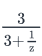=2 , y =

Solution:

We are given

2yz = 1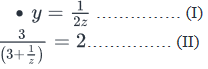Observe that there is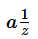in the denominator of (II).

From (I) we know that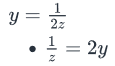Using this in (II) we get,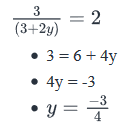QUESTION: 7

Charles spent \$28 in buying 4 cups of coffee, 2 burgers and 4 cones of ice cream. If the price of a cup of coffee is \$2 less than the price of an ice cream cone, and the price of a burger is half the price of an ice cream cone, what is the price of a burger (in dollars)?

Solution:

Let the price per unit of Coffee, Burger, and Ice Cream be C, B and R dollars respectively.

We need to find the value of B.

We are given that Charles spent \$28 to buy 4 cups of coffee, 2 burgers and 4 cones of ice cream. Therefore:

4C + 2B + 4R = 28  …………… (I)

We are also given Coffee costs \$2 less than Ice Cream. Therefore:

C = R - 2  …………….. (II)

The final piece of information given to us is that price of burger is half the price of ice cream.

B=R/2  ……………. (III)

Using (II) and (III) in (I), we get:

4(R−2)+2∗R/2+4R=28

• 4R−8+R+4R=28
• 9R=36
• R=4

Therefore from (III), we get

B=4/2

• B = 2

QUESTION: 8

A chef baked 12 cakes, of which some were strawberry-flavored and the others were chocolate-flavored. How many cakes were chocolate-flavored?

(1)   For every strawberry-flavored cake, two chocolate-flavored cakes were baked.

(2)   If both flavors of cakes were sold at \$2 per piece, a total revenue of \$24 was earned from the sale of the 12 cakes.

Solution:

Let’s say the number of strawberry flavored cakes and number of chocolate flavored cakes are S and C respectively.

⇒ S + C = 12 …………… (I)
We need to find the value of C
Step 1: Analyze Statement 1
Statement 1 says that for every strawberry-flavored cake, two chocolate-flavored cakes were baked.
This means:
if S straw-berry flavored cakes were baked, then 2S chocolate-flavored cakes would be baked.
Therefore, number of chocolate-flavored cakes, C = 2S
⇒ C = 2S ……………… (II)
Using (II) in (I), we get
S + 2S = 12
⇒ 3S = 12
⇒ S = 4
Therefore from (II)
C = 2 * 4
⇒ C = 8
Therefore statement 1 alone is sufficient to arrive at a unique answer.

Step 2: Analyze Statement 2
Statement 2 says that both varieties of cakes are sold at \$2 apiece and that the total revenue was \$24.
Therefore
2 * S + 2 * C = 24
⇒  2 * (S + C) = 24
⇒  S + C =12
But we already know this from (I).
Statement 2 doesn’t provide us with any new information.
So statement 2 is not sufficient to arrive at a unique answer.

QUESTION: 9

Jay’s age is twice the age of her daughter Mary today. Four years later, Jay's age will be twice the age of her son Hans at that time. If Jay is 20 years older than Hans today, what will be the age of Mary four years later?

Solution:

Let the current ages of Jay, Mary and Hans be J, M and H years respectively. We need to find the age of Mary after four years. In other words, we need to find the value of M+4

It is given that presently, Jay’s age is twice the age of Mary.

• J = 2M ……………….. (I)

It is also given that four years later, Jay’s age will be twice the age of Hans at that time.

• J + 4 = 2(H + 4)
• J + 4 = 2H + 8
• J - 2H - 4 = 0  ………….. (II)

The final piece of information given to us is that Jay is 20 years older than Hans today.

• J = H + 20
• H = J - 20  ……………….. (III)

Using (III) in (II), we get

J -2(J - 20) - 4 = 0

• J - 2J + 40 - 4 = 0
• J = 36

So we have the value of J. Now using this in (I), we get:

2M = J = 36

• M = 18

Therefore, M + 4 = 22

QUESTION: 10

if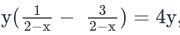and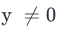, find the value of x.

Solution:

Step 1: Question statement and Inferences

Note that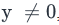which means we can divide both the sides of the equation by y. That would leave us with an equation in x alone, which we can solve.

Step 2: Finding required values

Given: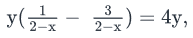Dividing both sides of the equation with y, we have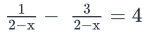Step 3: Finding the final answer